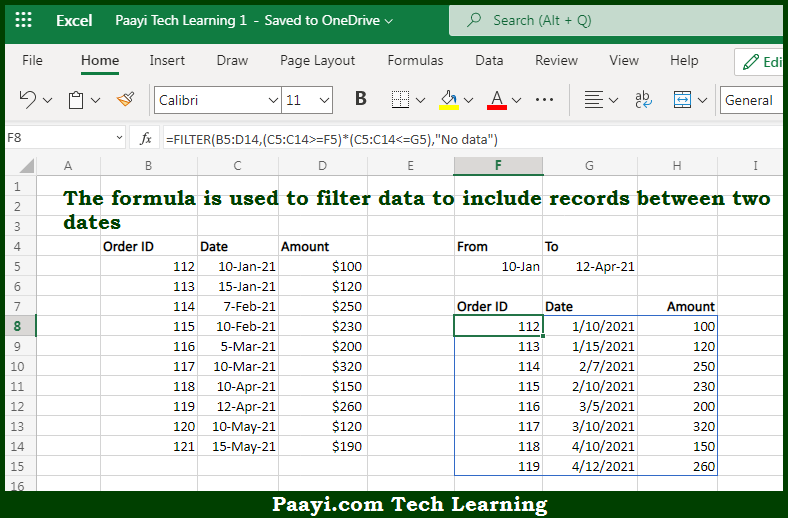# Learn How to Filter Data Between Dates in Microsoft Excel

Written by | 0 Comments | 647 Views

In this article, you will learn how to evaluate things in Dynamic Arrays with formulas in Microsoft Excel using a single/combination(s) of functions. You will also know How to Filter Data Between Dates and see the generic formula.

Learn How to Filter Data Between Dates in Microsoft Excel

The main purpose of this formula is to filter data to include records between two dates. Here we will learn how to filter data between dates in the given range in the workbook in Microsoft Excel. That implies, with the help of a formula based on the FILTER function you can able to filter data to include records between two dates. So, with the help of this formula, you can able to filter data between dates in the given range in the workbook in Microsoft Excel.

General Formula to Filter Data Between Dates

=FILTER(data,(dates>=A1)*(dates<=A2),"no data")

The Explanation for the Filter Data Between DatesSo we know that with the help of the given formula above you can able to filter data to include records between two dates. Here we will learn how to filter data between dates in the given range in the workbook in Microsoft Excel. As we know that the given formula relies on the FILTER function to retrieve data based on a logical test which is constructed with a Boolean logic expression. It should be noted that the array argument is provided in the B column, which contains the full set of data without headers. Moreover, the included argument is based on two logical comparisons. So, with the help of this formula, you can able to filter data to include records between two dates. Here we will learn how to filter data between dates in the given range in the workbook in Microsoft Excel.